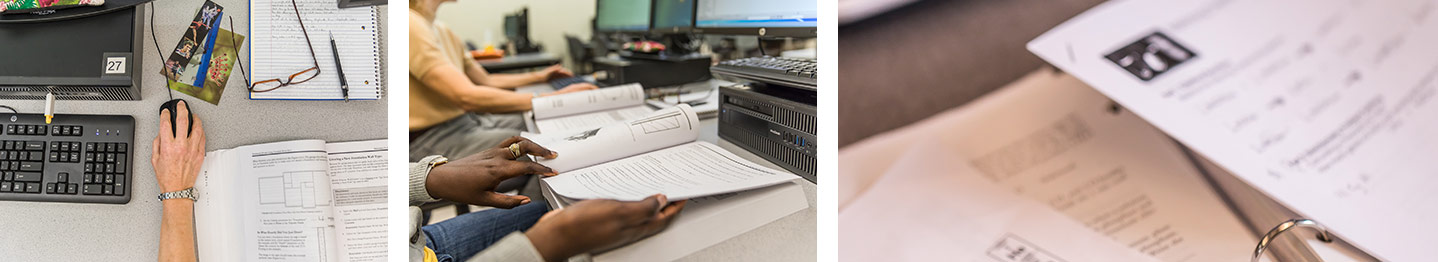Pre-College Mathematics
Program Learning Outcomes

Program Learning Outcomes detail the knowledge, skills and abilities that you as a student will demonstrate upon successful completion of your program.

#### Math, Developmental

1. In regards to solving problems, students will utilize various forms of validation, including the use of multiple strategies, recognizing the importance of evaluating solutions.

2. Students will be able to articulate their misunderstandings and problems in mathematics in order to improve their comprehension and meta-cognitive skills.

3. Students will work towards a mathematical tool box which includes a mastery of arithmetic, algebraic concepts, and the ability to create mathematical equations and models.

4. Students will be able to successfully manipulate algebraic expressions to solve multiple forms of equations.

5. Students will be able to connect mathematics skills from the classroom to real life problems in their lives.

6. Students will see value in mathematics and will leave the program with positive feelings about math.

Contact
Edgar Jasso
email
(206) 934-4516

Math & Science Division

Location
IB 2429

Division Contacts
(206) 934-3746
(206) 934-3748 (fax)